## Listening to the neural network gradient norms during training

Training neural networks is often done by measuring many different metrics such as accuracy, loss, gradients, etc. This is most of the time done aggregating these metrics and plotting visualizations on TensorBoard.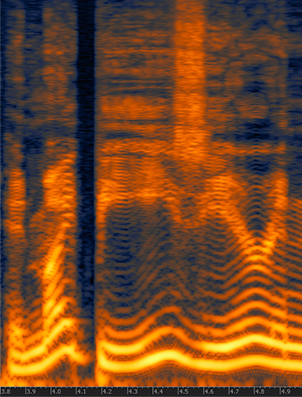There are, however, other senses that we can use to monitor the training of neural networks, such as sound. Sound is one of the perspectives that is currently very poorly explored in the training of neural networks. Human hearing can be very good a distinguishing very small perturbations in characteristics such as rhythm and pitch, even when these perturbations are very short in time or subtle.

For this experiment, I made a very simple example showing a synthesized sound that was made using the gradient norm of each layer and for step of the training for a convolutional neural network training on MNIST using different settings such as different learning rates, optimizers, momentum, etc.

You’ll need to install PyAudio and PyTorch to run the code (in the end of this post).

### Training sound with SGD using LR 0.01

This segment represents a training session with gradients from 4 layers during the first 200 steps of the first epoch and using a batch size of 10. The higher the pitch, the higher the norm for a layer, there is a short silence to indicate different batches. Note the gradient increasing during time.

### Training sound with SGD using LR 0.1

Same as above, but with higher learning rate.

### Training sound with SGD using LR 1.0

Same as above, but with high learning rate that makes the network to diverge, pay attention to the high pitch when the norms explode and then divergence.

### Training sound with SGD using LR 1.0 and BS 256

Same setting but with a high learning rate of 1.0 and a batch size of 256. Note how the gradients explode and then there are NaNs causing the final sound.

### Training sound with Adam using LR 0.01

This is using Adam in the same setting as the SGD.

## Source code

For those who are interested, here is the entire source code I used to make the sound clips:

import pyaudio
import numpy as np
import wave

import torch
import torch.nn as nn
import torch.nn.functional as F
import torch.optim as optim
from torchvision import datasets, transforms

class Net(nn.Module):
def __init__(self):
super(Net, self).__init__()
self.conv1 = nn.Conv2d(1, 20, 5, 1)
self.conv2 = nn.Conv2d(20, 50, 5, 1)
self.fc1 = nn.Linear(4*4*50, 500)
self.fc2 = nn.Linear(500, 10)

self.ordered_layers = [self.conv1,
self.conv2,
self.fc1,
self.fc2]

def forward(self, x):
x = F.relu(self.conv1(x))
x = F.max_pool2d(x, 2, 2)
x = F.relu(self.conv2(x))
x = F.max_pool2d(x, 2, 2)
x = x.view(-1, 4*4*50)
x = F.relu(self.fc1(x))
x = self.fc2(x)
return F.log_softmax(x, dim=1)

def open_stream(fs):
p = pyaudio.PyAudio()
stream = p.open(format=pyaudio.paFloat32,
channels=1,
rate=fs,
output=True)
return p, stream

def generate_tone(fs, freq, duration):
npsin = np.sin(2 * np.pi * np.arange(fs*duration) * freq / fs)
samples = npsin.astype(np.float32)
return 0.1 * samples

def train(model, device, train_loader, optimizer, epoch):
model.train()

fs = 44100
duration = 0.01
f = 200.0
p, stream = open_stream(fs)

frames = []

for batch_idx, (data, target) in enumerate(train_loader):
data, target = data.to(device), target.to(device)
output = model(data)
loss = F.nll_loss(output, target)
loss.backward()

norms = []
for layer in model.ordered_layers:

tone = f + ((norm_grad.numpy()) * 100.0)
tone = tone.astype(np.float32)
samples = generate_tone(fs, tone, duration)

frames.append(samples)

silence = np.zeros(samples.shape * 2,
dtype=np.float32)
frames.append(silence)

optimizer.step()

# Just 200 steps per epoach
if batch_idx == 200:
break

wf = wave.open("sgd_lr_1_0_bs256.wav", 'wb')
wf.setnchannels(1)
wf.setsampwidth(p.get_sample_size(pyaudio.paFloat32))
wf.setframerate(fs)
wf.writeframes(b''.join(frames))
wf.close()

stream.stop_stream()
stream.close()
p.terminate()

def run_main():
device = torch.device("cpu")

transform=transforms.Compose([
transforms.ToTensor(),
transforms.Normalize((0.1307,), (0.3081,))
])),
batch_size=256, shuffle=True)

model = Net().to(device)
optimizer = optim.SGD(model.parameters(), lr=0.01, momentum=0.5)

for epoch in range(1, 2):

if __name__ == "__main__":
run_main()


## The effective receptive field on CNNs

Given the interesting recent article on “The Emergence of a Fovea while Learning to Attend“, I decide to make a review of the paper written by Luo, Wenjie et al. called “Understanding the Effective Receptive Field in Deep Convolutional Neural Networks” where they introduced the idea of the “Effective Receptive Field” (ERF) and the surprising relationship with the foveal vision that arises naturally on Convolutional Neural Networks.

The receptive field in Convolutional Neural Networks (CNN) is the region of the input space that affects a particular unit of the network. Note that this input region can be not only the input of the network but also output from other units in the network, therefore this receptive field can be calculated relative to the input that we consider and also relative the unit that we are taking into consideration as the “receiver” of this input region. Usually, when the receptive field term is mentioned, it is taking into consideration the final output unit of the network (i.e. a single unit on a binary classification task) in relation to the network input (i.e. input image of the network).

It is easy to see that on a CNN, the receptive field can be increased using different methods such as: stacking more layers (depth), subsampling (pooling, striding), filter dilation (dilated convolutions), etc. In theory, when you stack more layers you can increase your receptive field linearly, however, in practice, things aren’t simple as we thought, as shown by Luo, Wenjie et al. article. In the article, they introduce the concept of the “Effective Receptive Field”, or ERF; the intuition behind the concept is that not all pixels in the receptive field contribute equally to the output unit’s response. When doing the forward pass, we can see that the central receptive field pixels can propagate their information to the output using many different paths, as they are part of multiple output unit’s calculations.

In the figure below, we can see in left the input pixels, after that we have a feature map calculated from the input pixels using a 3×3 convolution filter and then finally the output after another 3×3 filtering. The numbers inside the pixels on the left image represent how many times this pixel was part of a convolution step (each sliding step of the filter). As we can see, some pixels like the central ones will have their information propagated through many different paths in the network, while the pixels on the borders are propagated along a single path.

By looking at the image above, it isn’t that surprising that the effective receptive field impact on the final output computation will look more like a Gaussian distribution instead of a uniform distribution. What is actually more even interesting is that this receptive field is dynamic and changes during the training. The impact of this on the backpropagation is that the central pixels will have a larger gradient magnitude when compared to the border pixels.

In the article written by Luo, Wenjie et al., they devised a way to quantify the effect on each input pixel of the network by calculating the quantity$\frac{\partial y}{\partial x_{i, j}}$ that represents how much each pixel$x_{i, j}$ contributes to the output$y$.

In the paper, they did experimentations to visualize the effective receptive field using multiple different architectures, activations, etc. I replicate here the ones that I found most interesting: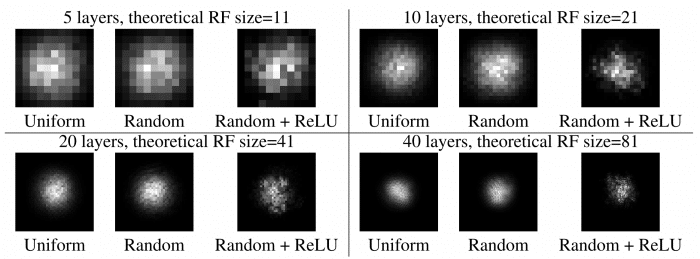Figure 1 from the paper “Understanding the Effective Receptive Field in Deep Convolutional Neural Networks”, by Luo, Wenjie et al.

As we can see from the Figure 1 of the paper, where they compare the effect of the number of layers, initialization schemes, and different activations, the results are amazing. We can clearly see the Gaussian and also the sparsity added by the ReLU activations.

There are also some comparisons on Figure 3 of the paper, where CIFAR-10 and CamVid datasets were used to train the network.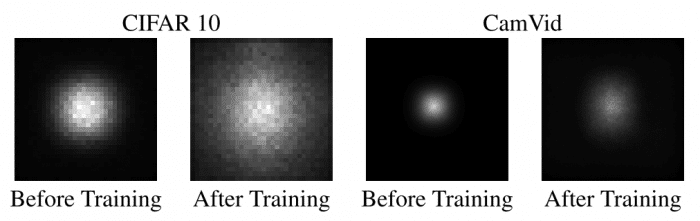Figure 3 of the paper “Understanding the Effective Receptive Field in Deep Convolutional Neural Networks”, by Luo, Wenjie et al.

As we can see, the size of the effective receptive field is very dynamic and it is increased by a large margin after the training, which implies, as stated by authors of the paper, that better initialization schemes can be employed to increase the receptive field in the beginning of the training. They actually developed a different initialization scheme and were able to get 30% training speed-up, however, these results weren’t consistent.

What is also very interesting, is that the effective receptive field has a very close relationship with the foveal vision of the human eye, which produces the sharp central vision, effect of the high-density region of cone cells (as shown in the image below) present in the eye fundus.

Our central sharp vision also decays rapidly like the effective receptive field that is very similar to a Gaussian. It is amazing that this effect is also naturally present on the CNN networks.

PS: Just for the sake of curiosity, some birds that do complex aerial movements such as the hummingbird, have two foveas instead of a single one, which means that they have a sharp accurate vision not only on the central region but also on the sides.

I hope you enjoyed the post !

– Christian S. Perone

Cite this article as: Christian S. Perone, "The effective receptive field on CNNs," in Terra Incognita, 12/11/2017, https://blog.christianperone.com/2017/11/the-effective-receptive-field-on-cnns/.

## Convolutional Neural Networks – Architectural Zoo

Presentation about an “Achitectural Zoo” of different applications and architectures of CNNs. Presented at Machine Learning Meetup in Porto Alegre yesterday.

Video (there are english subtitles available):

Cite this article as: Christian S. Perone, "Convolutional Neural Networks – Architectural Zoo," in Terra Incognita, 02/06/2016, https://blog.christianperone.com/2016/06/convolutional-neural-networks-architectural-zoo/.

## Convolutional hypercolumns in Python

If you are following some Machine Learning news, you certainly saw the work done by Ryan Dahl on Automatic Colorization (Hacker News comments, Reddit comments). This amazing work uses pixel hypercolumn information extracted from the VGG-16 network in order to colorize images. Samim also used the network to process Black & White video frames and produced the amazing video below:

Colorizing Black&White Movies with Neural Networks (video by Samim, network by Ryan)

But how does this hypercolumns works ? How to extract them to use on such variety of pixel classification problems ? The main idea of this post is to use the VGG-16 pre-trained network together with Keras and Scikit-Learn in order to extract the pixel hypercolumns and take a superficial look at the information present on it. I’m writing this because I haven’t found anything in Python to do that and this may be really useful for others working on pixel classification, segmentation, etc.

## Hypercolumns

Many algorithms using features from CNNs (Convolutional Neural Networks) usually use the last FC (fully-connected) layer features in order to extract information about certain input. However, the information in the last FC layer may be too coarse spatially to allow precise localization (due to sequences of maxpooling, etc.), on the other side, the first layers may be spatially precise but will lack semantic information. To get the best of both worlds, the authors of the hypercolumn paper define the hypercolumn of a pixel as the vector of activations of all CNN units “above” that pixel.

The first step on the extraction of the hypercolumns is to feed the image into the CNN (Convolutional Neural Network) and extract the feature map activations for each location of the image. The tricky part is when the feature maps are smaller than the input image, for instance after a pooling operation, the authors of the paper then do a bilinear upsampling of the feature map in order to keep the feature maps on the same size of the input. There are also the issue with the FC (fully-connected) layers, because you can’t isolate units semantically tied only to one pixel of the image, so the FC activations are seen as 1×1 feature maps, which means that all locations shares the same information regarding the FC part of the hypercolumn. All these activations are then concatenated to create the hypercolumn. For instance, if we take the VGG-16 architecture to use only the first 2 convolutional layers after the max pooling operations, we will have a hypercolumn with the size of:

64 filters (first conv layer before pooling)

+

128 filters (second conv layer before pooling ) = 192 features

This means that each pixel of the image will have a 192-dimension hypercolumn vector. This hypercolumn is really interesting because it will contain information about the first layers (where we have a lot of spatial information but little semantic) and also information about the final layers (with little spatial information and lots of semantics). Thus this hypercolumn will certainly help in a lot of pixel classification tasks such as the one mentioned earlier of automatic colorization, because each location hypercolumn carries the information about what this pixel semantically and spatially represents. This is also very helpful on segmentation tasks (you can see more about that on the original paper introducing the hypercolumn concept).

Everything sounds cool, but how do we extract hypercolumns in practice ?

## VGG-16

Before being able to extract the hypercolumns, we’ll setup the VGG-16 pre-trained network, because you know, the price of a good GPU (I can’t even imagine many of them) here in Brazil is very expensive and I don’t want to sell my kidney to buy a GPU.

from matplotlib import pyplot as plt

import theano
import cv2
import numpy as np
import scipy as sp

from keras.models import Sequential
from keras.layers.core import Flatten, Dense, Dropout
from keras.layers.convolutional import Convolution2D, MaxPooling2D
from keras.optimizers import SGD

from sklearn.manifold import TSNE
from sklearn import manifold
from sklearn import cluster
from sklearn.preprocessing import StandardScaler

def VGG_16(weights_path=None):
model = Sequential()

if weights_path:

return model

As you can see, this is a very simple code to declare the VGG16 architecture and load the pre-trained weights (together with Python imports for the required packages). After that we’ll compile the Keras model:

model = VGG_16('vgg16_weights.h5')
sgd = SGD(lr=0.1, decay=1e-6, momentum=0.9, nesterov=True)
model.compile(optimizer=sgd, loss='categorical_crossentropy')

Now let’s test the network using an image:

im_original = cv2.resize(cv2.imread('madruga.jpg'), (224, 224))
im = im_original.transpose((2,0,1))
im = np.expand_dims(im, axis=0)
im_converted = cv2.cvtColor(im_original, cv2.COLOR_BGR2RGB)
plt.imshow(im_converted)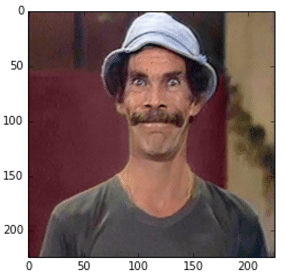Image used

As we can see, we loaded the image, fixed the axes and then we can now feed the image into the VGG-16 to get the predictions:

out = model.predict(im)
plt.plot(out.ravel())

As you can see, these are the final activations of the softmax layer, the class with the “jersey, T-shirt, tee shirt” category.

## Extracting arbitrary feature maps

Now, to extract the feature map activations, we’ll have to being able to extract feature maps from arbitrary convolutional layers of the network. We can do that by compiling a Theano function using the get_output() method of Keras, like in the example below:

get_feature = theano.function([model.layers.input], model.layers.get_output(train=False), allow_input_downcast=False)
feat = get_feature(im)
plt.imshow(feat)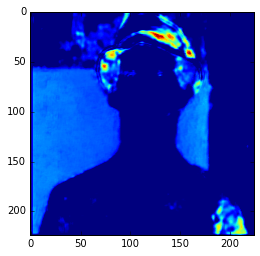Feature Map

In the example above, I’m compiling a Theano function to get the 3 layer (a convolutional layer) feature map and then showing only the 3rd feature map. Here we can see the intensity of the activations. If we get feature maps of the activations from the final layers, we can see that the extracted features are more abstract, like eyes, etc. Look at this example below from the 15th convolutional layer:

get_feature = theano.function([model.layers.input], model.layers.get_output(train=False), allow_input_downcast=False)
feat = get_feature(im)
plt.imshow(feat)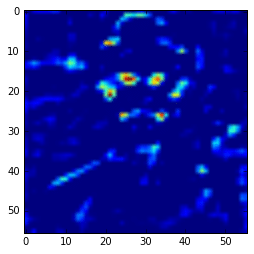More semantic feature maps.

As you can see, this second feature map is extracting more abstract features. And you can also note that the image seems to be more stretched when compared with the feature we saw earlier, that is because the the first feature maps has 224×224 size and this one has 56×56 due to the downscaling operations of the layers before the convolutional layer, and that is why we lose a lot of spatial information.

## Extracting hypercolumns

Now finally let’s extract the hypercolumns of arbitrary set of layers. To do that, we will define a function to extract these hypercolumns:

def extract_hypercolumn(model, layer_indexes, instance):
layers = [model.layers[li].get_output(train=False) for li in layer_indexes]
get_feature = theano.function([model.layers.input], layers,
allow_input_downcast=False)
feature_maps = get_feature(instance)
hypercolumns = []
for convmap in feature_maps:
for fmap in convmap:
upscaled = sp.misc.imresize(fmap, size=(224, 224),
mode="F", interp='bilinear')
hypercolumns.append(upscaled)

return np.asarray(hypercolumns)

As we can see, this function will expect three parameters: the model itself, an list of layer indexes that will be used to extract the hypercolumn features and an image instance that will be used to extract the hypercolumns. Let’s now test the hypercolumn extraction for the first 2 convolutional layers:

layers_extract = [3, 8]
hc = extract_hypercolumn(model, layers_extract, im)

That’s it, we extracted the hypercolumn vectors for each pixel. The shape of this “hc” variable is: (192L, 224L, 224L), which means that we have a 192-dimensional hypercolumn for each one of the 224×224 pixel (a total of 50176 pixels with 192 hypercolumn feature each).

Let’s plot the average of the hypercolumns activations for each pixel:

ave = np.average(hc.transpose(1, 2, 0), axis=2)
plt.imshow(ave)

Ad you can see, those first hypercolumn activations are all looking like edge detectors, let’s see how these hypercolumns looks like for the layers 22 and 29:

layers_extract = [22, 29]
hc = extract_hypercolumn(model, layers_extract, im)
ave = np.average(hc.transpose(1, 2, 0), axis=2)
plt.imshow(ave)


As we can see now, the features are really more abstract and semantically interesting but with spatial information a little fuzzy.

Remember that you can extract the hypercolumns using all the initial layers and also the final layers, including the FC layers. Here I’m extracting them separately to show how they differ in the visualization plots.

## Simple hypercolumn pixel clustering

Now, you can do a lot of things, you can use these hypercolumns to classify pixels for some task, to do automatic pixel colorization, segmentation, etc. What I’m going to do here just as an experiment, is to use the hypercolumns (from the VGG-16 layers 3, 8, 15, 22, 29) and then cluster it using KMeans with 2 clusters:

m = hc.transpose(1,2,0).reshape(50176, -1)
kmeans = cluster.KMeans(n_clusters=2, max_iter=300, n_jobs=5, precompute_distances=True)
cluster_labels = kmeans .fit_predict(m)

imcluster = np.zeros((224,224))
imcluster = imcluster.reshape((224*224,))
imcluster = cluster_labels

plt.imshow(imcluster.reshape(224, 224), cmap="hot")